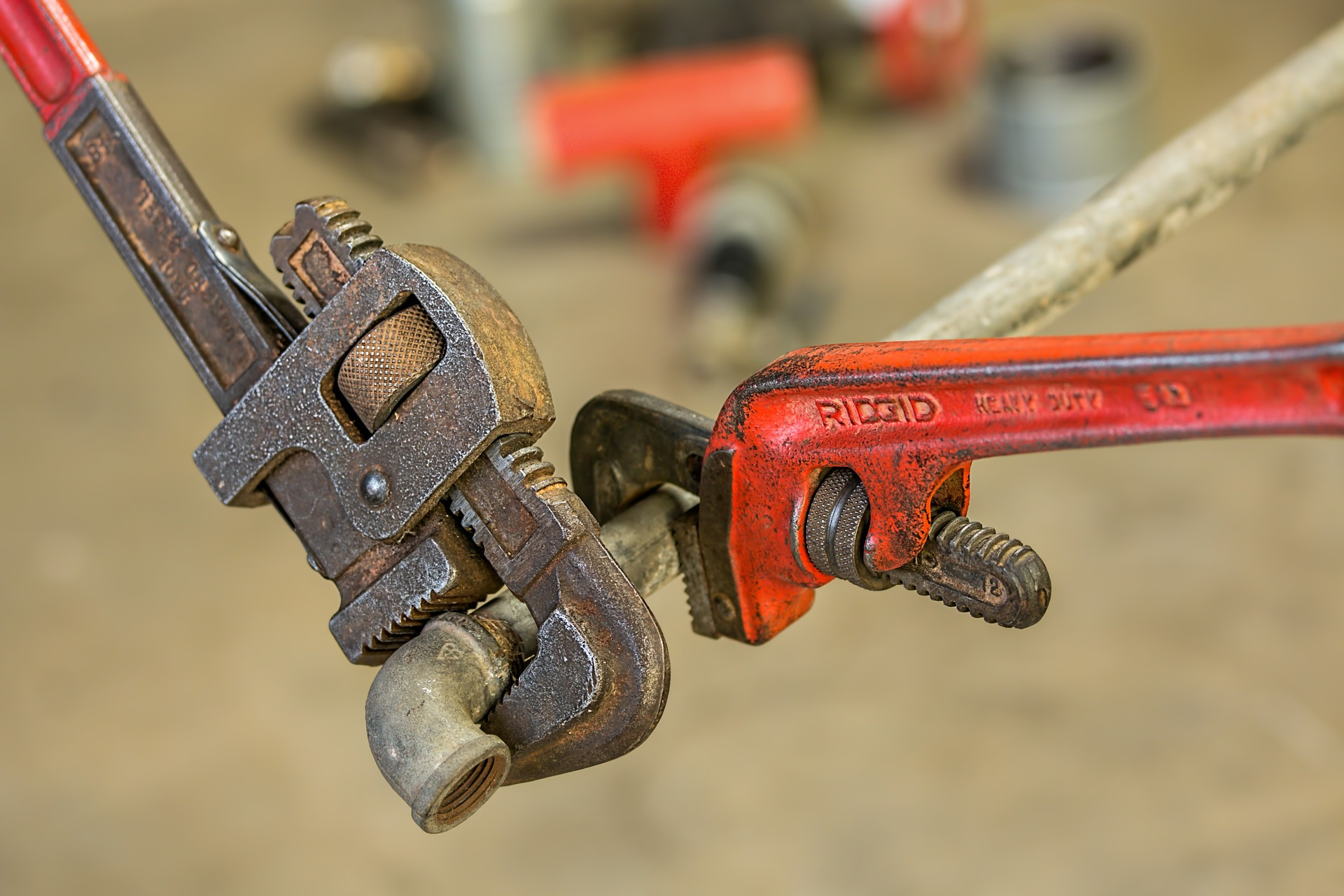# Characteristics of Piping Material

In previous post, we learned how select piping material. There are several considerations in selecting piping material, which are service life, code requirement, allowable stresses, design temperature, design pressure, corrosion, and economics. As a continuation of the post, we also need to understand characteristics of piping material. What are important characteristics of piping material?

Let’s find out.

The most important considerations in evaluating various pipe system materials are strength, ductility, toughness, and corrosion resistance. However, characteristics of piping material can be expressed by several parameters:

# Strength

Before talking about strength, there are two terminologies that we need to understand first: stress and strain.

Stress equal to force divided by the cross sectional area of the material. Strain is a change of the material per an original length.

Figure below illustrate the difference between stress and strain.

The strength of material can be defined by:

1. Modulus of elasticity (Young’s Modulus)
2. Yield strength
3. Ultimate tensile strength

To understand those terms, please see figure below.

Figure above is called stress-strain curve. It is derived from stress versus strain for a sample material.

## Modulus of Elasticity

Based on figure above, modulus of elasticity (Young’s Modulus) is the ratio of stress and strain along the axis. It is the slope of stress-strain curve within elastic zone.

Where the ratio is linear through a range of stress, the material is elastic. It means the material will return to its original once the applied stress is removed. If the material is loaded beyond the elastic range, it will begin to deform in a plastic manner.

## Yield Strength

Yield strength or yield point is the stress at which a material begins to plastically deform. Before the yield point the material will deform elastically and return to its original shape when the stress is released. If the tension applied over yield strength, the deformation will be permanent and non-reversible.

## Ultimate Strength

Ultimate strength is maximum stress applied before the material is completely parted. As the load increased beyond the yield strength, its cross-sectional area will decrease until the material cannot handle any further load increase.

# Ductility

Ductility is the amount of plastic deformation that a material undergoes in resisting the fracture under stress. Ductile metal will be easily shaped thus are cheaper to manufacture.

# Malleability

Malleability is the property of metal to be deformed by compression without cracking or rupturing. This property is useful for copper tubing which allow the tube to be bent quickly without expensive and time-consuming fittings.

# Hardness

Hardness is the property of being rigid and resistant to pressure.

# Brittleness

Brittleness is the tendency of metal to crack or break with deformation.

# Toughness

Toughness is the ability of metal to deform plastically and absorb energy in the process before fracturing. It is the indicator of how the given metal would fail at the application of stress beyond the capacity of the metal, and whether that failure will be ductile or brittle.

# Creep

Creep is progressive permanent deformation of material subjected to constant stress; also known as time dependent behaviour.

References:

Process Piping Material, CED Engineering.com